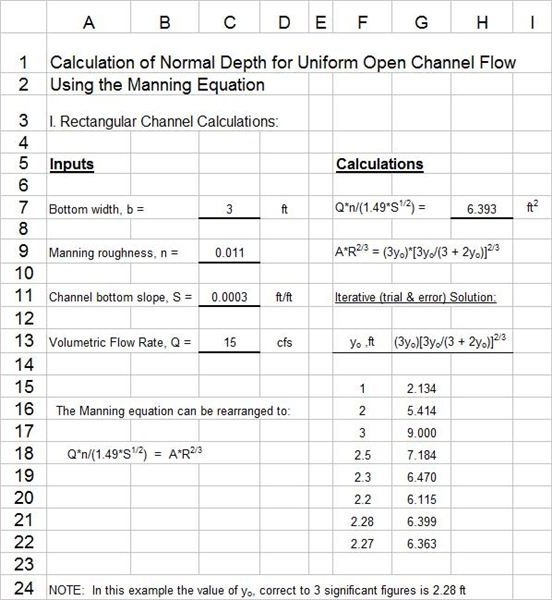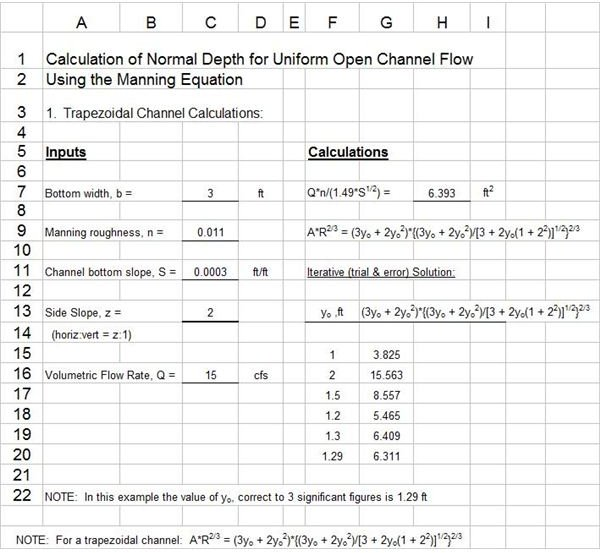# Use of Excel Spreadsheets for Manning Equation/Normal Depth Calculation with Open Channel Flow

## Calculating Open Channel Flow/Normal Depth

The normal depth for a reach of uniform open channel flow is the flow depth that will occur for a given volumetric flow rate in a channel of known bottom slope, shape, size, and Manning roughness. This calculation of normal depth using the Manning equation is an iterative process, and thus can be done well with Excel spreadsheets, like those that are available for download from this article. These Excel spreadsheet templates can make the normal depth calculations for an open channel of rectangular cross section and for an open channel of trapezoidal cross section.

## Excel Templates for Calculation of Normal Depth in a Rectangular ChannelThe Excel spreadsheet template at the left can be used to calculate the normal depth for uniform open channel flow in a rectangular channel. The channel width, b; Manning roughness coefficient, n; and bottom slope, S, must be specified along with the volumetric flow rate through the channel. Background information about the Manning equation [Q = (1.49/n)*A*R2/3*S1/2 ] is available in the article, "Introduction to the Manning Equation for Uniform Open Channel Flow Calculations."

The Manning Equation can be arranged into the form: Q*n/(1.49*S1/2) = A*R2/3, in which the left hand side of the equation is a constant that can be calculated from the specified values for Q, n, and S. The right hand side is a function of the normal depth yo. For a rectangular channel the right hand side of the equation becomes: A*R2/3 = (byo)*[byo/(b + 2yo)]2/3.

The last part of the Excel template is set up to facilitate the iterative calculations to find the value of yo that makes (byo)*[byo/(b + 2yo)]2/3 = Q*n/(1.49*S1/2).

## Excel Templates for Calculation of Normal Depth in a Trapezoidal Channel

The Excel spreadsheet template at the right can be used to calculate the normal depth for uniform open channel flow in a trapezoidal channel.In addition to the parameters in the previous section, a value is needed for the channel side slope, z, for a trapezoidal channel. For the trapezoidal channel, A*R2/3 = (b*yo + z*yo2)*{ (b*yo + z*yo2)/[ b + z*yo*(1 + z2) ]1/2 }2/3. The iterative procedure for calculating the normal depth is much the same as for the rectangular channel, but with a somewhat more complicated expression for A*R2/3.